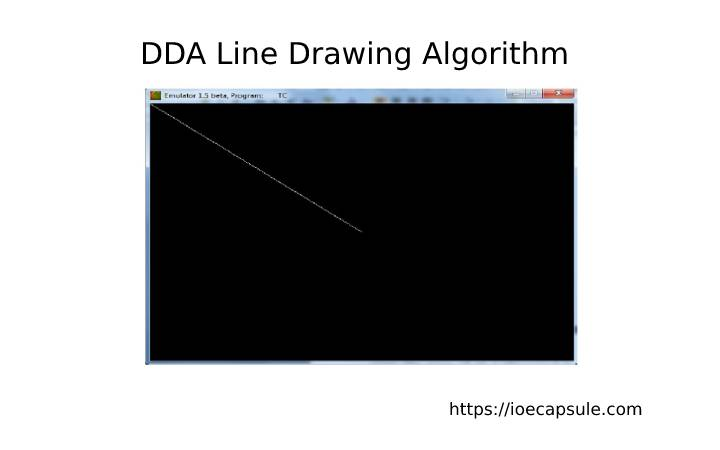### Computer Graphics: Digital Differential Analyzer (DDA) AlgorithmAlgorithms

Joining two points on any 2 dimensional plane results a line segment. Unfortunately, we can not obtain a line or join two co-ordinate points in Computer Graphics. What we can do is find the intermediate points between these two points and put a pixel for each intermediate points with a desired color.

The computer graphics function uses the output screen as a coordinate system. The TOP-LEFT corner is considered origin(0, 0). On moving right, the x-ordinate increases and on moving down, y-ordinate increases.

### What is DDA Algorithm ?

It is an algorithm for calculating all intermediate points in between two co-ordinate points in order to draw line between them.

### The DDA Line Drawing Algorithm

1. Start
2. Input line endpoints and store left and right endpoints in (x1, y1) and (x2, y2) respectively.
3. Calculate values of delta(x) and delta(y) :
delta(x) = x2 – x1
delta(y) = y2 – y1
4. if(abs(delta(x)) > abs(delta(y))) then
stepLength = abs(delta(x))
otherwise
stepLength = abs(delta(y))
5. Calculate the values of x-increment and y-increment :
xInc = delta(x)/stepLength
yInc = delta(y)/stepLength
6. Set x = x1, y = y1
7. Draw the pixel Round(x), Round(y)
8. For k = 0 to stepLength, do
x = x + xInc
y = y + yInc
draw Round(x), Round(y)
9. End

### C Implementation Of DDA Line Drawing Algorithm

``````
#include<stdio.h>
#include<graphics.h> //you need to have this library installed
#include<math.h>

void DDA(int x1, int y1, int x2, int y2)
{
int dx = x2 - x1;
int dy = y2 - y1;
int stepLength = abs(dx) > abs(dy) ? abs(dx) : abs(dy);
float xInc = dx / (float) stepLength;
float yInc = dy / (float) stepLength;

// Put pixel for each step
float x = x1;
float y = y1;
for (int i = 0; i <= steps; i++)
{
putpixel (x,y,RED);
x += xInc;
y += yInc;
}
}

int main()
{
int gd = DETECT, gm;
initgraph (&gd, &gm, "");
int x1 = 2, y1 = 2, x2 = 14, y2 = 16;
DDA(2, 2, 14, 16);
return 0;
}
``````

• It is the simplest method and involves only integer additions so easier to implement.
• It is the fastest method for calculating pixel positions then by direct user equation y = mx + c.

• Floating point arithmetic in DDA algorithm is time consuming.
• Rounding in DDA is also time consuming.
• The algorithm is orientation oriented thus the end point accuracy is poor.

### Example Problem Of DDA :

Digitize the line with end points (1, 5) and (7, 2) using DDA Algorithm.

solution : Here,

dx = 7 – 1 = 6

dy = 2 – 5 = -3

stepLength = 6 (since abs(dx) > abs(dy))

xInc = 6/6 = 1

yInc = -3/6 = -1/2 = -.5